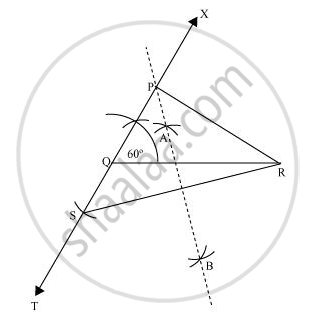Share

# Construct a triangle PQR in which QR = 6 cm, ∠Q = 60° and PR − PQ = 2 cm - CBSE Class 9 - Mathematics

ConceptSome Constructions of Triangles

#### Question

Construct a triangle PQR in which QR = 6 cm, ∠Q = 60° and PR − PQ = 2 cm

#### Solution

The below given steps will be followed to construct the required triangle.

Step I:- Draw line segment QR of 6 cm. At point Q, draw an angle of 60°, say ∠XQR.

Step II:- Cut a line segment QS of 2 cm from the line segment QT extended in the opposite side of line segment XQ. (As PR > PQ and PR - PQ = 2 cm). Join SR.

Step III:- Draw perpendicular bisector AB of line segment SR. Let it intersect QX at point P. Join PQ, PR.

ΔPQR is the required triangle.Is there an error in this question or solution?

#### APPEARS IN

NCERT Solution for Mathematics Class 9 (2018 to Current)
Chapter 11: Constructions
Ex. 11.20 | Q: 3 | Page no. 195

#### Video TutorialsVIEW ALL 

Solution Construct a triangle PQR in which QR = 6 cm, ∠Q = 60° and PR − PQ = 2 cm Concept: Some Constructions of Triangles.
S